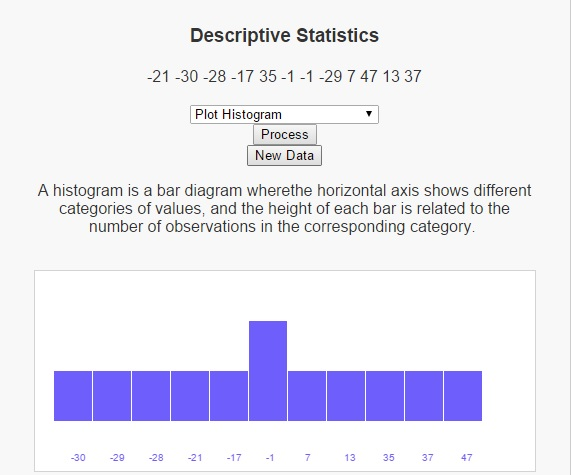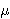# Tag Archives: meanI had been meaning to write a script and blog post on descriptive statistics for some time now, but with work and winter weather and the extra work that winter weather brings, and now that the winter weather is over trying to get back into an exercise routine (running up a hill is such a challenging experience, but when I get to the top of that hill I feel like Rocky Balboa on the steps at the steps at the entrance of the Philadelphia Museum of Art), I haven’t had the time to devote to this site that I would have liked. Well, that’s not entirely true. I have still been programming in my spare time. I just haven’t been able to share it here. I went to a conference in February and in my down time, I was able to write a script on descriptive statistics that I think gives a nice introduction to the area.

Before I go into descriptive statistics though, lets talk about statistics, which is concerned with the collection, analysis, interpretation and presentation of data. Statistics can generally be broken down into two categories, descriptive statistics and infernalinferential statistics, depending on what we would like to do with that data. When we are concerned with visualizing and summarizing the given data, descriptive statistics gives methods to operate on this data set. On the other hand, if we wish to draw conclusions about a larger population from our sample, then we would use methods from inferential statistics.

In the script on descriptive statistics I’ve written, I consider three different types of summaries for descriptive statistics:

Measures of Central Tendency
Mean – the arithmetic average of a set of values
Median – the middle number in a set of values
Mode – the most used number in a set of values

Dispersion
Maximum – the largest value in the data set
Minimum – the smallest value in the data set
Standard Deviation – the amount of variation in a set of data values
Variance – how far a set of numbers is spread out

Shape
Kurtosis – how peaked or flat a data set is
Skewness – how symmetric a data set is

Plots
Histogram Plots – a bar diagram where the horizontal axis shows different categories of values, and the height of each bar is related to the number of observations in the corresponding category.
Box and Whisker Plots – A box-and-whisker plot for a list of numbers consists of a rectangle whose left edge is at the first quartile of the data and whose right edge is at the third quartile, with a left whisker sticking out to the smallest value, and a right whisker sticking out to the largest value.
Stem and Leaf Plots – A stem and leaf plot illustrates the distribution of a group of numbers by arranging the numbers in categories based on the first digit.

# Covariance of VectorsMost of the things we think about have many different ways we could describe them. Take for example a movie. I could describe a movie by its genre, its length, the number of people in the movie, the number of award winners, the length of the explosions, the number of fight scenes, the number of scenes, the rating it was given by a certain critic, etc. The list goes on and on. How much do these things influence one another? How likely is a person to enjoy a movie? Is that related to the number of award winners in the movie? Answering this type of a question can often help understand things like what might influence a critics rating or more importantly which movies are worth my \$15 ticket price.

Movies are just one example of this. Other areas like sports, traffic congestion, or food and a number of others can be analyzed in a similar manner. With data becoming available at unprecedented rates and areas like cloud computing and data science becoming key buzzwords in industry, the ability to understand these relationships is becoming more and more important.

As a mathematician, I enjoy being able to say with certainty that some known truth is the cause of some other known truth, but it not always easy (or even possible) to prove the existence of such a relationship. We are left instead with looking at trends in data to see how similar things are to one another over a data set. Measuring the covariance of two or more vectors is one such way of seeking this similarity.

Before delving into covariance though, I want to give a refresher on some other data measurements that are important to understanding covariance.
– Sum of a vector:
If we are given a vector of finite length we can determine its sum by adding together all the elements in this vector. For example, consider the vector v = (1, 4, -3, 22). Then sum(v) = 1 + 4 + -3 + 22 = 24.

– Length of a vector:
If we are given a vector of finite length, we call the number of elements in the vector the length of the vector. So for the example above with the vector v = (1, 4, -3, 22), there are four elements in this vector, so length(v) = 4.

– Mean of a vector:
The mean of a finite vector is determined by calculating the sum and dividing this sum by the length of the vector. So, working with the vector above, we already calculated the sum as 24 and the length as 4, which we can use to calculate the mean as the sum divided by the length, or 24 / 4 = 6.

– Variance of a vector:
Once we know the mean of a vector, we are also interested in determining how the values of this vector are distributed across its domain. The variance measures this by calculating the average deviation from the mean. Here we calculate the deviation from the mean for the ith element of the vector v as (vi)2. We can get the average deviation from the mean then by computing the average of these values.

So if the vector v has n elements, then the variance of v can be calculated as Var(v) = (1/n)i = 1 to n((vi)2).

Once again dealing with the vector above with v = (1, 4, -3, 22), where the mean is 6, we can calculate the variance as follows:

 vi vi –(vi –)2 1 -5 25 4 -2 4 -3 -9 81 22 16 256

To calculate the mean of this new vector (25, 4, 81, 324), we first calculate the sum as 25 + 4 + 81 + 256 = 366. Since the length of the new vector is the same as the length of the original vector, 4, we can calculate the mean as 366 / 4 = 91.5

The covariance of two vectors is very similar to this last concept. Instead of being interested in how one vector is distributed across its domain as is the case with variance, covariance is interested in how two vectors X and Y of the same size are distributed across their respective means. What we are able to determine with covariance is things like how likely a change in one vector is to imply change in the other vector. Having a positive covariance means that as the value of X increases, so does the value of Y. Negative covariance says that as the value of X increases, the value of Y decreases. Having zero covariance means that a change in the vector X is not likely to affect the vector Y.

With that being said, here is the procedure for calculating the covariance of two vectors. Notice that it is very similar to the procedure for calculating the variance of two vectors described above. As I describe the procedure, I will also demonstrate each step with a second vector, x = (11, 9, 24, 4)

1. Calculate the means of the vectors.
As we’ve seen above, the mean of v is 6.
We can similarly calculate the mean of x as 11 + 9 + 24 + 4 = 48 / 4 = 12

2. Subtract the means of the vectors from each element of the vector (xiX) and (YiY).

We did this for v above when we calculated the variance. Below are the values for v and for x as well.

vi – meanv

-5

-2

-9

16

 i vi xi xi – meanx 1 1 11 -1 2 4 9 -3 3 -3 24 12 4 22 4 -8

3. For each element i, multiply the terms (xiX) and (YiY).

This gives us the following vector in our example:
(-5)(-1), (-2)(-3), (-9)(12), (16)(-8) = (5, 6, -108, -128).

4. Sum the elements obtained in step 3 and divide this number by the total number of elements in the vector X (which is equal to the number of elements in the vector Y).

When we sum the vector from step 3, we wind up with 5 + 6 + -108 + -128 = -225
And the result of dividing -225 by 4 gives us -225/4 = – 56.25.

This final number, which for our example is -56.25, is the covariance.

Some important things to note are

• If the covariance of two vectors is positive, then as one variable increases, so does the other.
• If the covariance of two vectors is negative, then as one variable increases, the other decreases.
• If the covariance of two vectors is 0, then one variable increasing (decreasing) does not impact the other.
• The larger the absolute value of the covariance, the more often the two vectors take large steps at the same time.
• A low covariance does not necessarly mean that the two variables are independent. I’ll give a quick example to illustrate that.
Consider the vectors x and y given by x = (-3, -2, -1, 0, 1, 2, 3) and y = (9, 4, 1, 0, 1, 4, 9).
The mean of x is 0, while the mean of y is 7.
The mean adjusted values are (-3, -2, -1, 0, 1, 2, 3) and (2, -3, -6, -7, -6, -3, 2).
The product of these mean adjusted values is (-6, 6, 6, 0, -6, -6, 6).
If we sum this last vector, we get 0, which after dividing by 7 still gives a value of 0.
So the covariance of these two vectors is 0.

We can easily see that for each value xi in x, the corresponding yi is equal to xi2

I have written a script to help understand the calculation of two vectors.